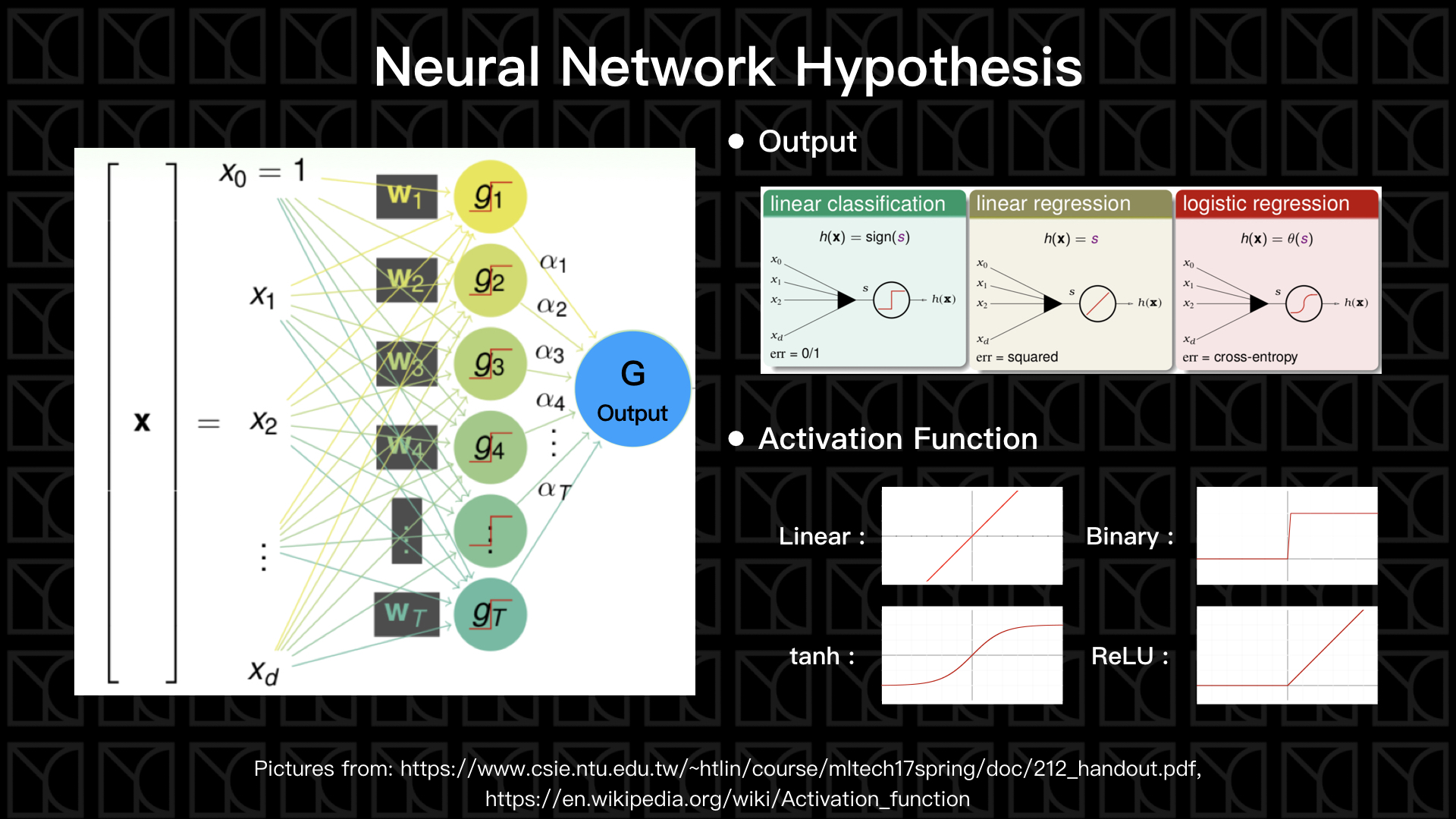# 機器學習技法 學習筆記 (6)：神經網路(Neural Network)與深度學習(Deep Learning)

### 神經網路(Neural Network)

Extraction Models的基本款就是廣為人知的「神經網路」(Neural Network)，它的特色是使用神經元來做非線性的特徵轉換，那如果具有多層神經元，就是做了多次的非線性特徵轉換，這就是所謂的「深度學習」(Deep Learning)。### 深度學習(Deep Learning)

Neural Network的歷史相當悠久，早在1958年就有人提出以Perceptron當作Activation Function的單層Neural Network，大家也知道一層的Neural Network是不Powerful的，所以在1969年，就有人寫了論文叫做「perceptron has limitation」，從那時起Neural Network的方法就很少人研究了。

### 反向傳播算法(Backpropagation, BP)1. 定義出Error函數
2. Error函數讓我們可以去評估$$E_{in}$$
3. 算出它的梯度$$∇E_{in}$$
4. 朝著$$∇E_{in}$$的反方向更新參數W，而每次只跨出$$η$$大小的一步
5. 反覆的計算新參數$$W$$的梯度，並一再的更新參數$$W$$

$$L = (1/2) (y-\overline{y})^{2}$$

$$W_{ij}^{(ℓ)} ← W_{ij}^{(ℓ)}-η×∂L/∂W_{ij}^{(ℓ)}$$

$$∂L/∂W_{ij}^{(L)}$$

$$= \frac{∂L}{∂s_{j}^{(L)}} \frac{∂s_{j}^{(L)}}{{∂W_{ij}^{(L)}}}$$ (連鎖率)

$$= {δ_{j}^{(L)}}×{X_{i}^{(L-1)}}$$

$$δ_{j}^{(L)} = ∂L/∂s_{j}^{(L)}$$

$$δ_{j}^{(L)}$$這一項被稱為Backward Pass Term，而$$X_{i}^{(L-1)}$$這項被稱為Forward Pass Term，所以$$L$$層權重的更新取決於Forward Pass Term和Backward Pass Term相乘$$δ_{j}^{(L)}×X_{i}^{(L-1)}$$

$$δ_{j}^{(L)} = ∂L/∂s_{j}^{(L)} = ∂[(1/2) (y-\overline{y})^{2}]/∂y = (y-\overline{y})$$

$$W_{ij}^{(L)} ← W_{ij}^{(L)}-η×δ_{j}^{(L)}×X_{i}^{(L-1)}$$

$$∂L/∂W_{ij}^{(ℓ)}$$

$$= \frac{∂L}{∂s_{j}^{(ℓ)}}\frac{∂s_{j}^{(ℓ)}}{∂W_{ij}^{(ℓ)}}$$ (連鎖率)

$$= δ_{j}^{(ℓ)}×X_{i}^{(ℓ-1)}$$

$$δ_{j}^{(ℓ)}$$

$$= ∂L/∂s_{j}^{(ℓ)}$$

$$= 𝚺_{k} \frac{∂L}{∂s_{k}^{(ℓ+1)}}\frac{∂s_{k}^{(ℓ+1)}}{∂X_{jk}^{(ℓ)}}\frac{∂X_{jk}^{(ℓ)}}{∂s_{j}^{(ℓ)}}$$ (連鎖率)

$$= 𝚺_{k} {δ_{k}^{(ℓ+1)}}×{W_{jk}^{(ℓ)}}×{σ'(s_{j}^{(ℓ)})}$$

$$W_{jk}^{(ℓ)}$$$$σ'(s_{j}^{(ℓ)})$$都是Forward之後就會得到的資訊，而$$δ_{k}^{(ℓ+1)}$$ 而是需要Backward才可以得到，我們已經知道$$δ_{j}^{(ℓ=L)}$$的值，就可以從$$δ_{j}^{(ℓ=L)}$$開始利用上面的公式，一路Backward把所有的$$δ_{j}$$都找齊。好！那現在我們已經找到了更新所有Weights的方法了。

$$W_{ij}^{(ℓ)} ← W_{ij}^{(ℓ)}-η×δ_{j}^{(ℓ)}×X_{i}^{(ℓ-1)}$$

If output layer ($$ℓ=L$$), $$δ_{j}^{(ℓ=L)}=(y-ŷ)$$

If other layer, $$δ_{j}^{(ℓ)}= σ'(s_{j}^{(ℓ)}) × 𝚺_{k} δ_{k}^{(ℓ+1)}×W_{jk}^{(ℓ)}$$

$$δ_{j}^{(ℓ)}$$為Backword Pass Term；$$X_{i}^{(ℓ-1)}$$為Forward Pass Term。

### Regularization in Deep Learning

Weight-elimination L2 regularizer: $$𝚺\frac{(W_{jk}^{(ℓ)})^{2}}{1+(W_{jk}^{(ℓ)})^{2}}$$

### AutoencoderNeural Network針對不同需要發展出很多不同的型態，包括CNN, RNN，還有接下來要介紹的Autoencoder，Autoencoder是一種可以將資料重要資訊保留下來的Neural Network，效果有點像是資料壓縮，在做資料壓縮時，會有一個稱為Encoder的方法可以將資料壓縮，那當然還要有另外一個方法將它還原回去，這方法稱為Decoder，壓縮的過程就是用更精簡的方式保存了資料。Autoencoder同樣的有Encoder和Decoder，不過它不像資料壓縮一樣可以百分之一百還原，不過特別之處是Autoencoder會試著從Data中自己學習出Encoder和Decoder，並盡量讓資料在壓縮完了可以還原回去原始數據

Encoder這一段就是在做一個Demension Reduction，Encoder轉換原本數據到一個新的空間，這個空間可以比原本Features描述的空間更能精準的描述這群數據，而中間這層Layer的數值就是新空間裡頭的座標，有些時候我們會用這個新空間來判斷每筆Data之間彼此的接近程度。

### Principal Component Analysis (PCA)PCA的演算法是這樣的，第一步先求出資料Features的平均值，並且將各個Features減掉平均值，令為$$ζ$$，第二步求出由$$ζ^{T}ζ$$產生的矩陣的Eigenvalue和Eigenvector，第三步，從這些Eigenvalue和Eigenvector中挑選前面$$k$$個，並組成轉換矩陣$$W$$，而最終PCA的轉換就是$$Φ(x)=W^{T}(X-mean(X))$$，這個轉換做的就是Dimension Reduction，將數據降維到$$k$$維。

PCA做的事是這樣的，每一個Eigenvector代表新空間裡頭的一個軸，而Eigenvalue代表站在這個軸上看資料的離散程度，當然我們如果可以描述每筆資料越分離，就代表這樣的描述方法越好，所以Eigenvalue越大的Eigenvector越是重要，所以取前面$$k$$個Eigenvector的用意是在降低維度的過程，還可以盡量的保持對數據的描述力，而且Eigenvector彼此是正交的，也就是說在新空間裡頭的每個軸是彼此垂直，彼此沒有Dependent的軸是最精簡的，所以PCA所做的Dimension Reduction一定是線性模型中最好、最有效率的